In grade 4, students work with customary and metric units as they practice converting measurements between different units of the same system. We’ve segmented our measurement section by length, weight, capacity, temperature, mixed units and word problems worksheets.

In this section, students use their understanding of customary and metric units of length when measuring objects, read rulers, they compare lengths and convert units.

This worksheet covers the reading of rulers.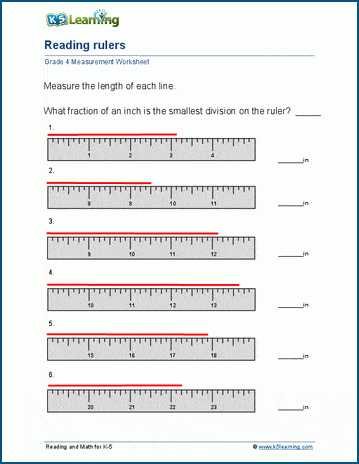## Weight worksheets for grade 4 students

Similar to the length section, the weight section provides worksheets on understanding customary and metric units when weighing objects, reading and balancing scales, comparing and converting weights.

Here, students balance scales.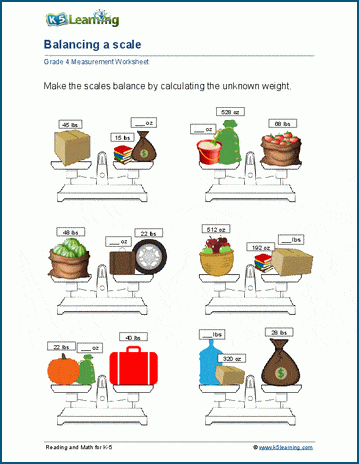## Capacity practice for grade 4

Students start by using their understanding of units of capacity to measuring objects, then move onto capacity charts, reading measuring cups, comparing capacities and converting between different capacity units.

This capacity worksheet has students create their own capacity charts by drawing equivalent measures.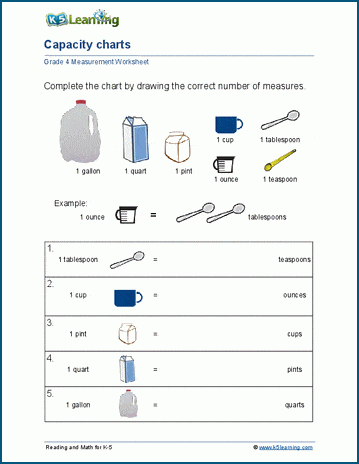## Temperature worksheets

We have two sets of worksheets in this area: understanding Fahrenheit and Celsius, as well as converting between Fahrenheit and Celsius.

This is what the converting temperatures worksheet looks like: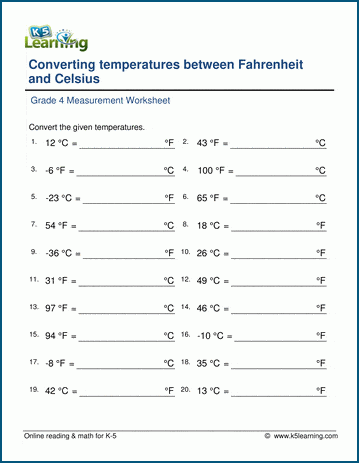## Practice mixed units at the grade 4 level

As the title suggests, this area contains worksheets of mixed units of measurement, including estimating measurements, converting mixed units. There are also worksheets on metric abbreviations and measurement projects.

Students pick real life objects and measure them by length, capacity and weight in these worksheets. Here’s the lengths measurement worksheet: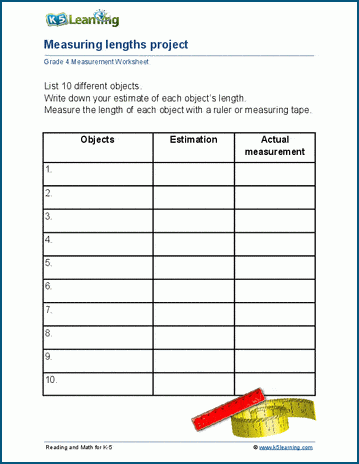## Grade 4 measurement word problems

The final section covers word problems: length, mass and weight, volume and capacity, money and time word problems to be exact.

This worksheet covers mass and weight word problems.